Updating search results...

# 24 Results

View
Selected filters:
• CCSS.Math.Content.7.NS.A.1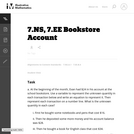Unrestricted Use
CC BY
Rating
0.0 stars

This is a task from the Illustrative Mathematics website that is one part of a complete illustration of the standard to which it is aligned. Each task has at least one solution and some commentary that addresses important asects of the task and its potential use. Here are the first few lines of the commentary for this task: At the beginning of the month, Evan had \24 in his account at the school bookstore. Use a variable to represent the unknown quantity in each transacti... Subject: Mathematics Material Type: Activity/Lab Provider: Illustrative Mathematics Provider Set: Illustrative Mathematics Author: Illustrative Mathematics Date Added: 05/13/2013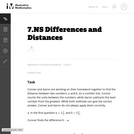Unrestricted Use CC BY Rating 0.0 stars This is a task from the Illustrative Mathematics website that is one part of a complete illustration of the standard to which it is aligned. Each task has at least one solution and some commentary that addresses important asects of the task and its potential use. Here are the first few lines of the commentary for this task: Conner and Aaron are working on their homework together to find the distance between two numbers,a$and$b, on a number line. Conner count the units... Subject: Mathematics Material Type: Activity/Lab Provider: Illustrative Mathematics Provider Set: Illustrative Mathematics Author: Illustrative Mathematics Date Added: 09/07/2013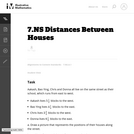Unrestricted Use CC BY Rating 0.0 stars This is a task from the Illustrative Mathematics website that is one part of a complete illustration of the standard to which it is aligned. Each task has at least one solution and some commentary that addresses important asects of the task and its potential use. Here are the first few lines of the commentary for this task: Aakash, Bao Ying, Chris and Donna all live on the same street as their school, which runs from east to west. Aakash lives5 \frac{1}{2}blocks to the... Subject: Mathematics Material Type: Activity/Lab Provider: Illustrative Mathematics Provider Set: Illustrative Mathematics Author: Illustrative Mathematics Date Added: 05/16/2013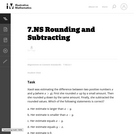Unrestricted Use CC BY Rating 0.0 stars This is a task from the Illustrative Mathematics website that is one part of a complete illustration of the standard to which it is aligned. Each task has at least one solution and some commentary that addresses important asects of the task and its potential use. Here are the first few lines of the commentary for this task: Xiaoli was estimating the difference between two positive numbersx$and$y$(where$x\gt y$). First she rounded$x\$ up by a small amount. Then she ro...

Subject:
Mathematics
Material Type:
Activity/Lab
Provider:
Illustrative Mathematics
Provider Set:
Illustrative Mathematics
Author:
Illustrative Mathematics
05/14/2013Conditional Remix & Share Permitted
CC BY-NC-SA
Rating
0.0 stars

Subject:
Mathematics
Material Type:
Activity/Lab
Assessment
Author:
Liberty Public Schools
04/08/2021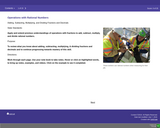Only Sharing Permitted
CC BY-NC-ND
Rating
0.0 stars

Students learn how to add, subtract, multiply, & divide fractions and decimals.

Subject:
Mathematics
Numbers and Operations
Material Type:
Activity/Lab
Assessment
Homework/Assignment
Author:
Rob Robinson
07/09/2019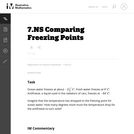Unrestricted Use
CC BY
Rating
0.0 stars

This task is appropriate for assessing student's understanding of differences of signed numbers. Because the task asks how many degrees the temperature drops, it is correct to say that "the temperature drops 61.5 degrees." However, some might think that the answer should be that the temperature is "changing -61.5" degrees. Having students write the answer in sentence form will allow teachers to interpret their response in a way that a purely numerical response would not.

Subject:
Mathematics
Material Type:
Activity/Lab
Provider:
Illustrative Mathematics
Provider Set:
Illustrative Mathematics
Author:
Illustrative Mathematics
05/01/2012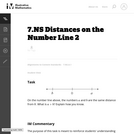Unrestricted Use
CC BY
Rating
0.0 stars

The purpose of this task is meant to reinforce students' understanding of rational numbers as points on the number line and to provide them with a visual way of understanding that the sum of a number and its additive inverse (usually called its "opposite") is zero.

Subject:
Mathematics
Material Type:
Activity/Lab
Provider:
Illustrative Mathematics
Provider Set:
Illustrative Mathematics
Author:
Illustrative Mathematics
05/01/2012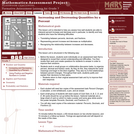Only Sharing Permitted
CC BY-NC-ND
Rating
0.0 stars

This lesson unit is intended to help teachers assess how well students are able to interpret percent increase and decrease, and in particular, to identify and help students who have the following difficulties: translating between percents, decimals, and fractions; representing percent increase and decrease as multiplication; and recognizing the relationship between increases and decreases.

Subject:
Mathematics
Ratios and Proportions
Material Type:
Assessment
Lesson Plan
Provider:
Shell Center for Mathematical Education
Provider Set:
Mathematics Assessment Project (MAP)
04/26/2013Conditional Remix & Share Permitted
CC BY-NC
Rating
0.0 stars

Four full-year digital course, built from the ground up and fully-aligned to the Common Core State Standards, for 7th grade Mathematics. Created using research-based approaches to teaching and learning, the Open Access Common Core Course for Mathematics&nbsp;is designed with student-centered learning in mind, including activities for students to develop valuable 21st century skills and academic mindset.

Subject:
Mathematics
Material Type:
Full Course
Provider:
Pearson
10/06/2016Conditional Remix & Share Permitted
CC BY-NC
Rating
0.0 stars

Working With Rational Numbers

Type of Unit: Concept

Prior Knowledge

Students should be able to:

Compare and order positive and negative numbers and place them on a number line.
Understand the concepts of opposites absolute value.

Lesson Flow

The unit begins with students using a balloon model to informally explore adding and subtracting integers. With the model, adding or removing heat represents adding or subtracting positive integers, and adding or removing weight represents adding or subtracting negative integers.

Students then move from the balloon model to a number line model for adding and subtracting integers, eventually extending the addition and subtraction rules from integers to all rational numbers. Number lines and multiplication patterns are used to find products of rational numbers. The relationship between multiplication and division is used to understand how to divide rational numbers. Properties of addition are briefly reviewed, then used to prove rules for addition, subtraction, multiplication, and division.

This unit includes problems with real-world contexts, formative assessment lessons, and Gallery problems.

Subject:
Algebra
Mathematics
Material Type:
Unit of Study
Provider:
PearsonConditional Remix & Share Permitted
CC BY-NC
Rating
0.0 stars

Subject:
Numbers and Operations
Material Type:
Lesson Plan
09/21/2015Conditional Remix & Share Permitted
CC BY-NC
Rating
0.0 stars

Students use the Hot Air Balloon simulation to model integer subtraction. They then move to modeling subtraction on a number line. They use patterns in their work and their answers to write a rule for subtracting integers.Key ConceptsThis lesson introduces the number line model for subtracting integers. To subtract on a number line, start at 0. Move to the location of the first number (the minuend). Then, move in the negative direction (down or left) to subtract a positive integer or in the positive direction (up or right) to subtract a negative integer. In other words, to subtract a number, move in the opposite direction than you would if you were adding it.The Hot Air Balloon simulation can help students see why subtracting a number is the same as adding the opposite:Subtracting a positive number means removing heat from air, which causes the balloon to go down, in the negative direction.Subtracting a negative number means removing weight, which causes the balloon to go up, in the positive direction.The rule for integer subtraction (which extends to addition of rational numbers) is easiest to state in terms of addition: to subtract a number, add its opposite. For example, 5 &ndash; 2 = 5 + (&ndash;2) = 3 and 5 &ndash; (&ndash;2) = 5 + 2 = 7.Goals and Learning ObjectivesModel integer subtraction on a number line.Write a rule for subtracting integers.

Subject:
Numbers and Operations
Material Type:
Lesson Plan
09/21/2015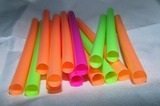Conditional Remix & Share Permitted
CC BY-NC
Rating
0.0 stars

Subject:
Numbers and Operations
Material Type:
Lesson Plan
09/21/2015Conditional Remix & Share Permitted
CC BY-NC
Rating
0.0 stars

Students critique and improve their work on the Self Check, then work on more addition and subtraction problems.Students solve problems that require them to apply their knowledge of adding and subtracting positive and negative numbers.Key ConceptsTo solve the problems in this lesson, students use their knowledge of addition and subtraction with positive and negative numbers.Goals and Learning ObjectivesUse knowledge of addition and subtraction with positive and negative numbers to write problems that meet given criteria.Assess and critique methods for subtracting negative numbers.Find values of variables that satisfy given inequalities.

Subject:
Numbers and Operations
Material Type:
Lesson Plan
09/21/2015Conditional Remix & Share Permitted
CC BY-NC
Rating
0.0 stars

Subject:
Numbers and Operations
Material Type:
Lesson Plan
09/21/2015Conditional Remix & Share Permitted
CC BY-NC
Rating
0.0 stars

Students find the distance between points on a number line by counting and by using subtraction. They then use subtraction to find differences in temperatures.Students discover that the distance between any two points on the number line is the absolute value of their difference, and apply this idea to solve problems.Key ConceptsStudents know from earlier grades that the distance between two positive numbers on the number line can be found by subtracting the lesser number from the greater number. For example, the distance between 5 and 11 is 11 &ndash; 5, or 6. We can also state the rule for finding distance as &ldquo;The distance between two positive numbers is the absolute value of their difference.&rdquo; With this version of the rule, we don&rsquo;t have to consider which number is greater; the result is the same either way. Using the example of 5 and 11, the distance is |11 &ndash; 5| or |5 &ndash; 11|, both of which are equal to 6.This idea extends to the entire number line, including numbers to the left of 0. That is, the distance between any two numbers is the absolute value of their difference. For example, the distance between &ndash;5 and 3 is |&ndash;5 &ndash; 3| = |&ndash;8| = 8 or |3 &ndash; (&ndash;5)| = |8| = 8, and the distance between &ndash;12 and &ndash;7 is |&ndash;12 &ndash; (&ndash;7)| = |&ndash;5| = 5 or |&ndash;7 &ndash; (&ndash;12)| = |5| = 5.Goals and Learning ObjectivesUnderstand the relationship between the distance between two points on the number line and the difference in the coordinates of those points.Find distances in real-life situations.

Subject:
Numbers and Operations
Material Type:
Lesson Plan
09/21/2015Conditional Remix & Share Permitted
CC BY-NC
Rating
0.0 stars

Students use number lines to solve addition and subtraction problems involving positive and negative fractions and decimals. They then verify that the same rules they found for integers apply to fractions and decimals as well. Finally, they solve some real-world problems.Key ConceptsThe first four lessons of this unit focused on adding and subtracting integers. Using only integers made it easier for students to create models and visualize the addition and subtraction process. In this lesson, those concepts are extended to positive and negative fractions and decimals. Students will see that the number line model and rules work for these numbers as well.Note that rational number will be formally defined in Lesson 15.Goals and Learning ObjectivesExtend models and rules for adding and subtracting integers to positive and negative fractions and decimals.Solve real-world problems involving addition and subtraction of positive and negative fractions and decimals.

Subject:
Numbers and Operations
Material Type:
Lesson Plan
09/21/2015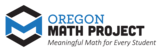Unrestricted Use
CC BY
Rating
0.0 stars

The intent of clarifying statements is to provide additional guidance for educators to communicate the intent of the standard to support the future development of curricular resources and assessments aligned to the 2021 math standards.&nbsp; Clarifying statements can be in the form of succinct sentences or paragraphs that attend to one of four types of clarifications: (1) Student Experiences; (2) Examples; (3) Boundaries; and (4) Connection to Math Practices.

Subject:
Mathematics
Material Type:
Teaching/Learning Strategy
Author:
Mark Freed
07/10/2023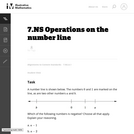Unrestricted Use
CC BY
Rating
0.0 stars

The purpose of this task is to help solidify students' understanding of signed numbers as points on a number line and to understand the geometric interpretation of adding and subtracting signed numbers.

Subject:
Mathematics
Material Type:
Activity/Lab
Provider:
Illustrative Mathematics
Provider Set:
Illustrative Mathematics
Author:
Illustrative Mathematics